Sara Stoudt true
04-16-2018

# Setup

``````require(readxl)
require(dplyr)
require(ggplot2)
require(gridExtra)
require(tidyr)
require(RColorBrewer)
``````

# Week 3 - Global causes of mortality

``````setwd("~/Desktop/tidytuesday/data/2018/2018-04-16")

gm.gathered <- gather(gm, cause, percent, -country, -country_code, -year) ## want a single column for cause of death
gm.gathered\$cause <- as.vector(gsub(" \\(\\%\\)", "", gm.gathered\$cause)) ## remove (%) in causes of death
``````

I will want the color per cause to be the same across plots.

The colors I use are still not perfectly distinguishable. Any suggestions?

``````colorOrder <- colorRampPalette(c("red", "orange", "yellow", "green", "blue", "purple"))(length(unique(gm.gathered\$cause)))
colorOrderShuffle <- colorOrder[sample(1:length(colorOrder), length(colorOrder))]
## don't want causes close in alphabetical order to be near the same color mainly because of prevalence of
## cancer and cardiovascular diseases

colorMap <- cbind.data.frame(
colorOrderShuffle,
unique(gm.gathered\$cause)
)
names(colorMap) <- c("color", "cause")
``````

# Plot Function

Here is a function to, given a country, plot the causes of death that have the top N highest average percentage and coefficients of variation across the time span.

``````makePlotTopN <- function(data, Country, N) {
dataToUse <- subset(data, country == Country) ## get country of interest

byCause <- group_by(dataToUse, cause) %>% summarise(avgPercent = mean(percent), sdPercent = sd(percent), sdPercentNorm = sd(percent) / avgPercent)
## find average and standard deviation of percentages of causes of death across the time frame

byCauseM <- byCause %>% arrange(desc(avgPercent))
byCauseSD <- byCause %>% arrange(desc(sdPercentNorm))

toPlotM <- subset(dataToUse, cause %in% byCauseM\$cause[1:N]) ## get top N average
toPlotSD <- subset(dataToUse, cause %in% byCauseSD\$cause[1:N]) ## get top N variability

## want colors to be the same across plots
## is there an easier way?
toPlotM\$cause <- as.factor(toPlotM\$cause)
toPlotSD\$cause <- as.factor(toPlotSD\$cause)

toMergeM <- as.data.frame(toPlotM\$cause)
toMergeSD <- as.data.frame(toPlotSD\$cause)
names(toMergeM) <- names(toMergeSD) <- "cause"

col1 <- unique(merge(toMergeM, colorMap, by.x = "cause", by.y = "cause"))
col2 <- unique(merge(toMergeSD, colorMap, by.x = "cause", by.y = "cause"))

## plots
g1 <- ggplot(toPlotM, aes(x = year, y = percent, color = cause)) +
geom_line(size = 2) +
ggtitle(paste(Country, ": Top ", N, " Highest Average Cause of Death")) +
ylab("Percentage of Deaths") +
xlab("Year") +
scale_colour_manual(values = as.character(col1\$color))

g2 <- ggplot(toPlotSD, aes(x = year, y = percent, color = cause)) +
geom_line(size = 2) +
ggtitle(paste(Country, ": Top ", N, " Highest Coeff of Var Cause of Death")) +
ylab("Percentage of Deaths") +
xlab("Year") +
scale_colour_manual(values = as.character(col2\$color))

grid.arrange(g1, g2, ncol = 2)
}
``````

Following the article to choose sample countries.

``````makePlotTopN(gm.gathered, "United States", 10)
``````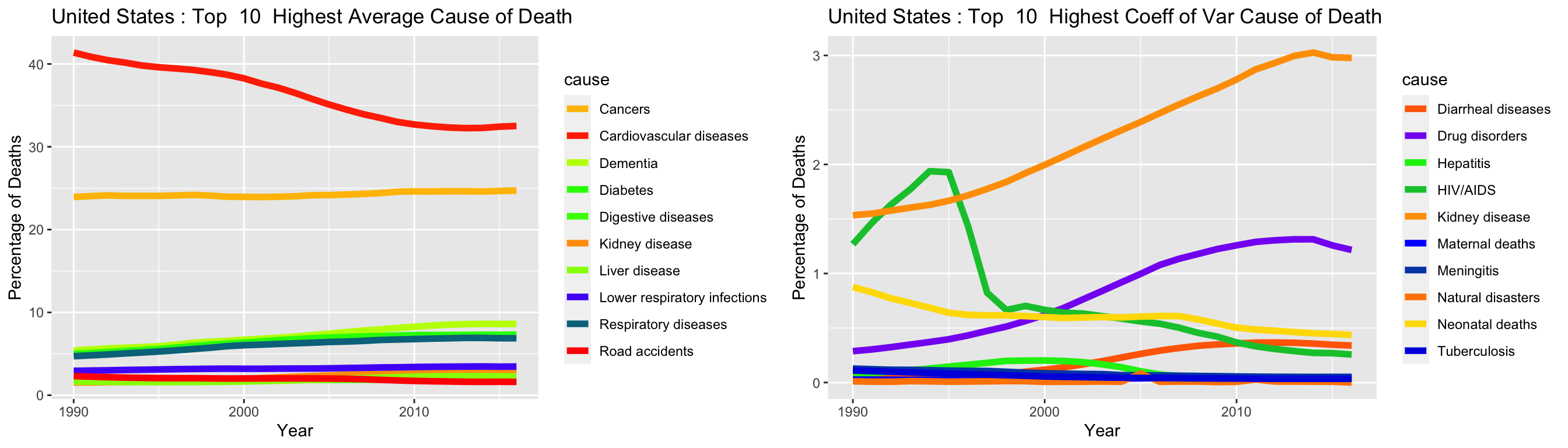``````makePlotTopN(gm.gathered, "Germany", 10)
``````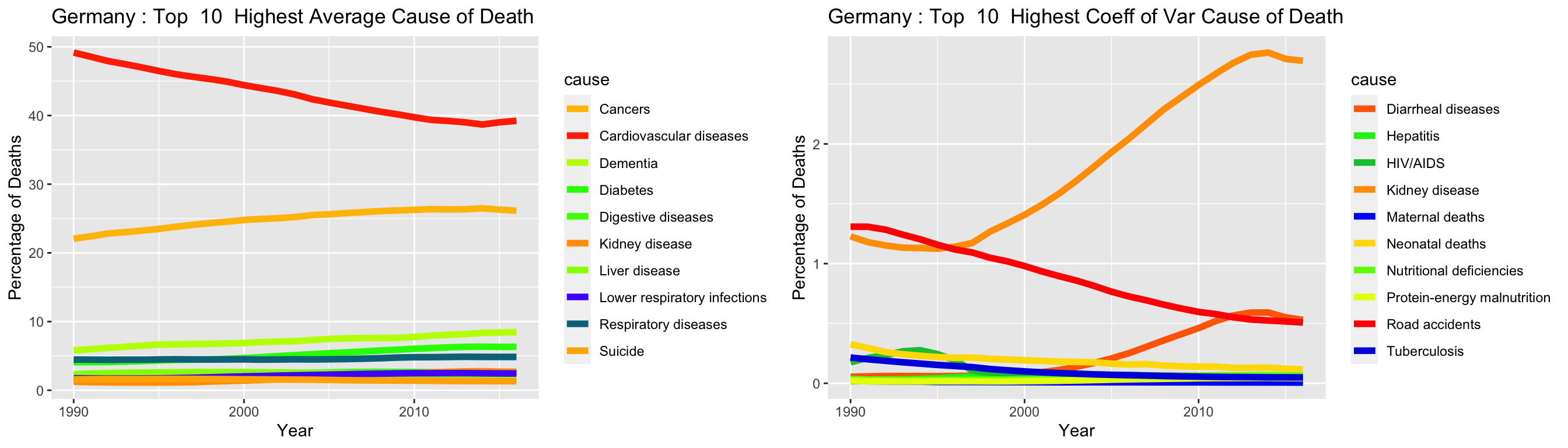``````makePlotTopN(gm.gathered, "Brazil", 10)
````````````makePlotTopN(gm.gathered, "South Africa", 10)
``````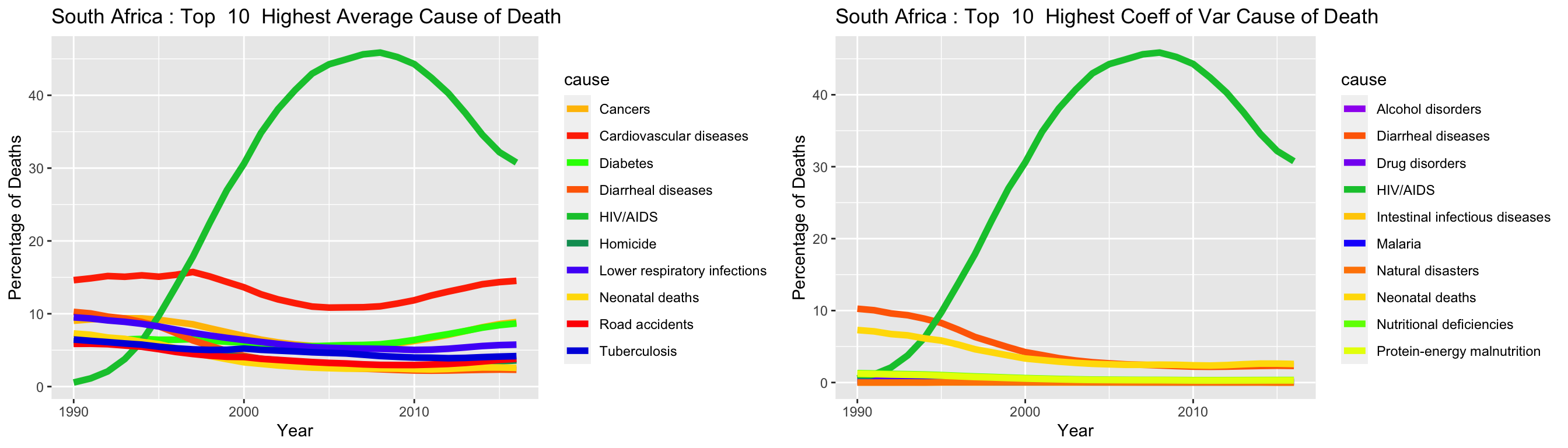``````makePlotTopN(gm.gathered, "Kenya", 10)
``````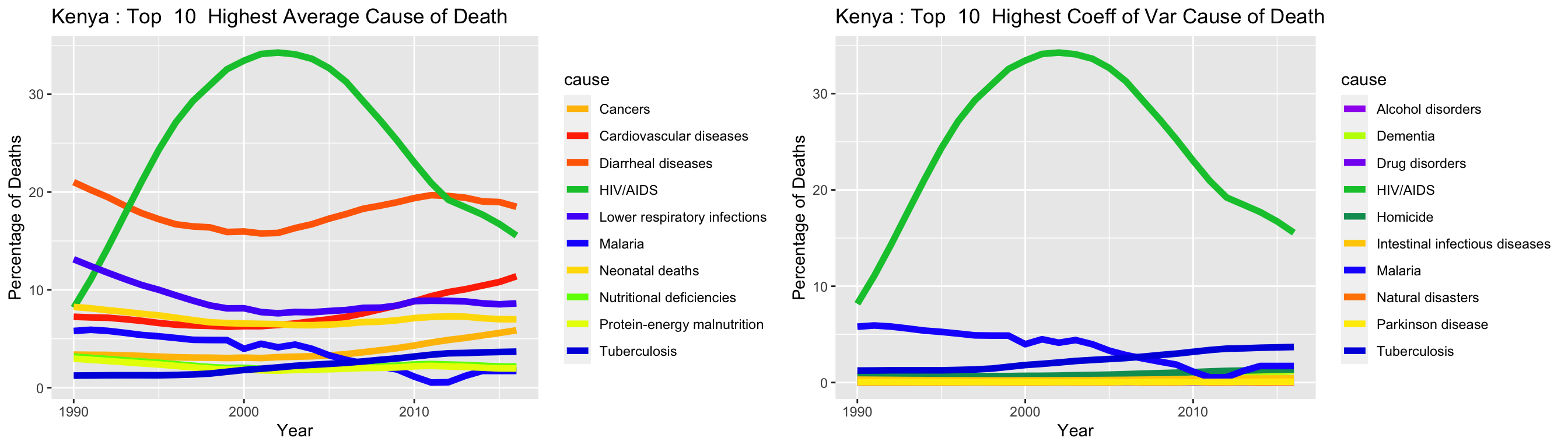``````makePlotTopN(gm.gathered, "Iraq", 10)
``````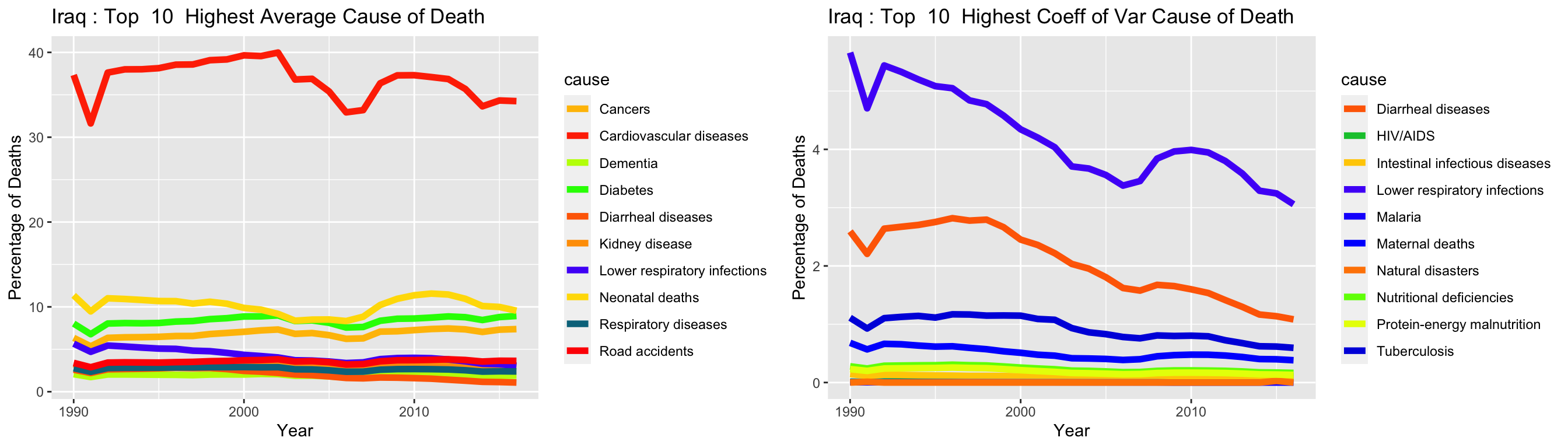Note: I wondered how @dpseidel had her Tidy Tuesday plots displayed on Github and discovered this.